# sklearn.linear_model.LinearRegression¶

class sklearn.linear_model.LinearRegression(*, fit_intercept=True, copy_X=True, n_jobs=None, positive=False)[source]

Ordinary least squares Linear Regression.

LinearRegression fits a linear model with coefficients w = (w1, …, wp) to minimize the residual sum of squares between the observed targets in the dataset, and the targets predicted by the linear approximation.

Parameters:
fit_interceptbool, default=True

Whether to calculate the intercept for this model. If set to False, no intercept will be used in calculations (i.e. data is expected to be centered).

copy_Xbool, default=True

If True, X will be copied; else, it may be overwritten.

n_jobsint, default=None

The number of jobs to use for the computation. This will only provide speedup in case of sufficiently large problems, that is if firstly n_targets > 1 and secondly X is sparse or if positive is set to True. None means 1 unless in a joblib.parallel_backend context. -1 means using all processors. See Glossary for more details.

positivebool, default=False

When set to True, forces the coefficients to be positive. This option is only supported for dense arrays.

New in version 0.24.

Attributes:
coef_array of shape (n_features, ) or (n_targets, n_features)

Estimated coefficients for the linear regression problem. If multiple targets are passed during the fit (y 2D), this is a 2D array of shape (n_targets, n_features), while if only one target is passed, this is a 1D array of length n_features.

rank_int

Rank of matrix X. Only available when X is dense.

singular_array of shape (min(X, y),)

Singular values of X. Only available when X is dense.

intercept_float or array of shape (n_targets,)

Independent term in the linear model. Set to 0.0 if fit_intercept = False.

n_features_in_int

Number of features seen during fit.

New in version 0.24.

feature_names_in_ndarray of shape (n_features_in_,)

Names of features seen during fit. Defined only when X has feature names that are all strings.

New in version 1.0.

Ridge

Ridge regression addresses some of the problems of Ordinary Least Squares by imposing a penalty on the size of the coefficients with l2 regularization.

Lasso

The Lasso is a linear model that estimates sparse coefficients with l1 regularization.

ElasticNet

Elastic-Net is a linear regression model trained with both l1 and l2 -norm regularization of the coefficients.

Notes

From the implementation point of view, this is just plain Ordinary Least Squares (scipy.linalg.lstsq) or Non Negative Least Squares (scipy.optimize.nnls) wrapped as a predictor object.

Examples

>>> import numpy as np
>>> from sklearn.linear_model import LinearRegression
>>> X = np.array([[1, 1], [1, 2], [2, 2], [2, 3]])
>>> # y = 1 * x_0 + 2 * x_1 + 3
>>> y = np.dot(X, np.array([1, 2])) + 3
>>> reg = LinearRegression().fit(X, y)
>>> reg.score(X, y)
1.0
>>> reg.coef_
array([1., 2.])
>>> reg.intercept_
3.0...
>>> reg.predict(np.array([[3, 5]]))
array([16.])


Methods

 fit(X, y[, sample_weight]) Fit linear model. get_params([deep]) Get parameters for this estimator. Predict using the linear model. score(X, y[, sample_weight]) Return the coefficient of determination of the prediction. set_params(**params) Set the parameters of this estimator.
fit(X, y, sample_weight=None)[source]

Fit linear model.

Parameters:
X{array-like, sparse matrix} of shape (n_samples, n_features)

Training data.

yarray-like of shape (n_samples,) or (n_samples, n_targets)

Target values. Will be cast to X’s dtype if necessary.

sample_weightarray-like of shape (n_samples,), default=None

Individual weights for each sample.

New in version 0.17: parameter sample_weight support to LinearRegression.

Returns:
selfobject

Fitted Estimator.

get_params(deep=True)[source]

Get parameters for this estimator.

Parameters:
deepbool, default=True

If True, will return the parameters for this estimator and contained subobjects that are estimators.

Returns:
paramsdict

Parameter names mapped to their values.

predict(X)[source]

Predict using the linear model.

Parameters:
Xarray-like or sparse matrix, shape (n_samples, n_features)

Samples.

Returns:
Carray, shape (n_samples,)

Returns predicted values.

score(X, y, sample_weight=None)[source]

Return the coefficient of determination of the prediction.

The coefficient of determination $$R^2$$ is defined as $$(1 - \frac{u}{v})$$, where $$u$$ is the residual sum of squares ((y_true - y_pred)** 2).sum() and $$v$$ is the total sum of squares ((y_true - y_true.mean()) ** 2).sum(). The best possible score is 1.0 and it can be negative (because the model can be arbitrarily worse). A constant model that always predicts the expected value of y, disregarding the input features, would get a $$R^2$$ score of 0.0.

Parameters:
Xarray-like of shape (n_samples, n_features)

Test samples. For some estimators this may be a precomputed kernel matrix or a list of generic objects instead with shape (n_samples, n_samples_fitted), where n_samples_fitted is the number of samples used in the fitting for the estimator.

yarray-like of shape (n_samples,) or (n_samples, n_outputs)

True values for X.

sample_weightarray-like of shape (n_samples,), default=None

Sample weights.

Returns:
scorefloat

$$R^2$$ of self.predict(X) w.r.t. y.

Notes

The $$R^2$$ score used when calling score on a regressor uses multioutput='uniform_average' from version 0.23 to keep consistent with default value of r2_score. This influences the score method of all the multioutput regressors (except for MultiOutputRegressor).

set_params(**params)[source]

Set the parameters of this estimator.

The method works on simple estimators as well as on nested objects (such as Pipeline). The latter have parameters of the form <component>__<parameter> so that it’s possible to update each component of a nested object.

Parameters:
**paramsdict

Estimator parameters.

Returns:
selfestimator instance

Estimator instance.

## Examples using sklearn.linear_model.LinearRegression¶Principal Component Regression vs Partial Least Squares Regression

Principal Component Regression vs Partial Least Squares Regression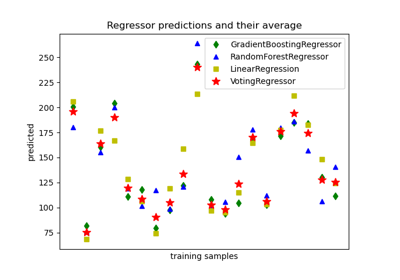Plot individual and voting regression predictions

Plot individual and voting regression predictions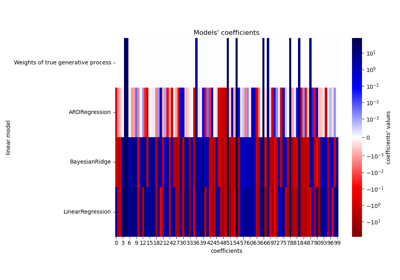Comparing Linear Bayesian Regressors

Comparing Linear Bayesian RegressorsLinear Regression Example

Linear Regression ExampleLogistic function

Logistic function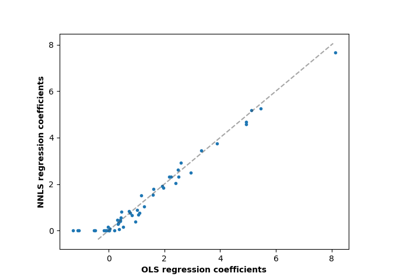Non-negative least squares

Non-negative least squaresOrdinary Least Squares and Ridge Regression Variance

Ordinary Least Squares and Ridge Regression Variance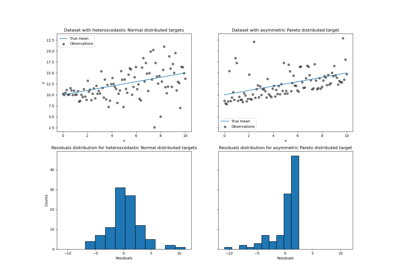Quantile regression

Quantile regression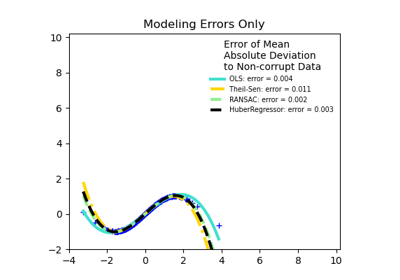Robust linear estimator fitting

Robust linear estimator fitting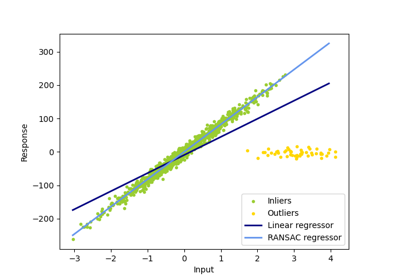Robust linear model estimation using RANSAC

Robust linear model estimation using RANSACSparsity Example: Fitting only features 1 and 2

Sparsity Example: Fitting only features 1 and 2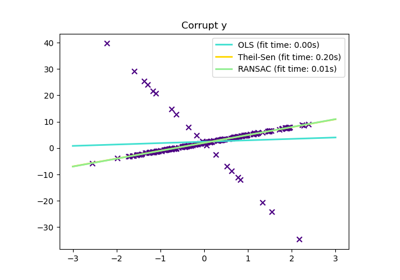Theil-Sen Regression

Theil-Sen Regression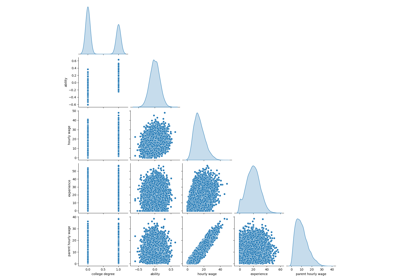Failure of Machine Learning to infer causal effects

Failure of Machine Learning to infer causal effects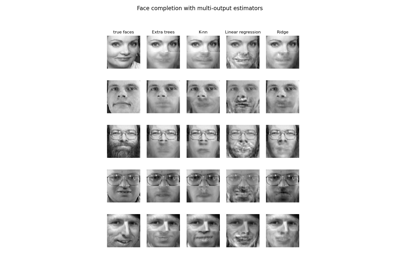Face completion with a multi-output estimators

Face completion with a multi-output estimators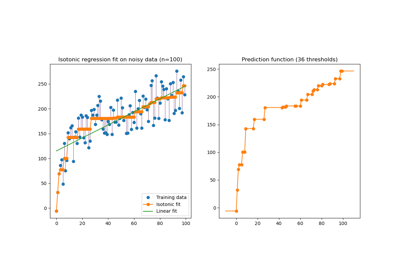Isotonic Regression

Isotonic Regression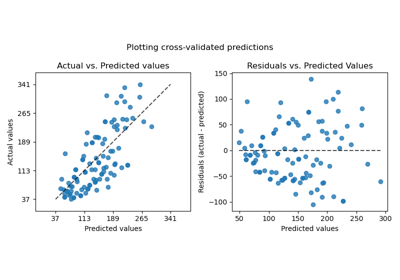Plotting Cross-Validated Predictions

Plotting Cross-Validated Predictions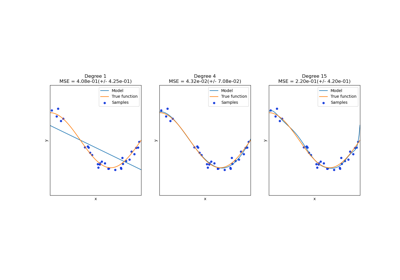Underfitting vs. Overfitting

Underfitting vs. Overfitting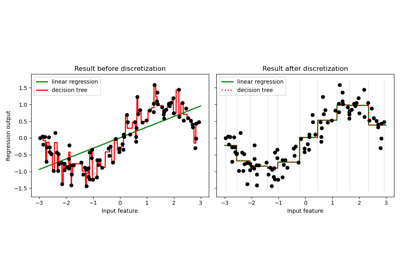Using KBinsDiscretizer to discretize continuous features

Using KBinsDiscretizer to discretize continuous features